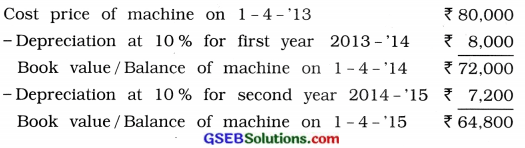# GSEB Solutions Class 11 Accounts Part 2 Chapter 2 Depreciation Accounts

Gujarat Board GSEB Textbook Solutions Class 11 Commerce Accounts Part 2 Chapter 2 Depreciation Accounts Textbook Exercise Questions and Answers.

## Gujarat Board Textbook Solutions Class 11 Accounts Part 2 Chapter 2 Depreciation Accounts

### GSEB Class 11 Accounts Depreciation Accounts Text Book Questions and Answers

Question 1.
Write correct option from those given below each question:

1. Depreciation is what kind of expense of business?
(a) Capital
(b) Revenue
(c) Capital and Revenue
(d) Fictitious
(b) Revenue

2. On which price of asset, depreciation is calculated under straight-line method?
(a) Book value
(b) Depreciated price
(c) Selling price
(d) Cost price
(d) Cost price

3. Depreciation indicates ……………….. in useful value of assets.
(a) increase
(b) reduction
(c) increase-reduction
(d) none of these
(b) Reduction

4. Under which method the annual amount of depreciation remains identical?
(a) Straight-line method
(b) Reducing balance method
(c) Revaluation method
(d) Mileage method.
(a) Straight-line method5. Which of the following asset is not depreciable?
(a) Building
(b) Furniture
(c) Machines
(d) Land
(d) Land

6. Under which method of depreciation, value of asset never becomes zero?
(a) Reducing balance method
(b) Annuity method
(c) Depletion unit method
(d) Equal instalment method
(a) Reducing balance method

7. Under which method of depreciation, balance is maintained between depreciation amount and repairing expense?
(a) Machine hour method
(b) Compound interest method
(c) Reducing balance method
(d) Straight-line method
(c) Reducing balance method

8. To which of the following depreciation is applicable?
(a) Capital
(b) Sales
(c) Liabilities
(d) None of these
(a) Capital

9. Which of the following expense is not paid in cash?
(a) Rent
(b) Commission
(c) Salary
(d) Depreciation
(d) Depreciation

10. Where does the loss on sale of machine will be recorded?
(a) Credit side of machine account
(b) Debit side of machine account
(c) Credit side of depreciation account
(d) Debit side of depreciation account
(a) Credit side of machine accountQuestion 2.
Answer the following questions in one sentence:

1. Under which method annual amount of depreciation reduces every year?
Under Reducing Balance Method, annual amount of depreciation reduces every year.

2. What are depreciable assets?
There is reduction in value of some assets due to their limited life and used for more than one accounting year, they are called as depreciable assets.

3. Explain the meaning of depreciation.
Depreciation means a gradual and permanent reduction in the value of an asset due to any reason.

4. On which assets of business, depreciation is calculated?
Depreciation is calculated on the fixed tangible asset of a business.

5. Write journal entry to transfer depreciation to profit and loss account at the end of the year.
Profit and Loss A/c Dr …………………………
To Depreciation A/c …………………….
(Being amount of depreciation transferred to Profit and Loss A/c after closing the Depreciation A/c.)

6. Write the formula to determine the depreciation rate under equal instalment method.
Rate of depreciation R = $$\frac{D}{C} \times 100$$ Where, D = Annual depreciation
C = Cost price of an asset

7. Describe different methods to record depreciation on assets from the viewpoint of accounting entries.
From the viewpoint of accounting entries, there are two main methods of recording depreciation on assets :

• Method of providing depreciation on the respective assets.
• Method of provision for depreciation.8. Which account is credited to record depreciation under depreciation provision method?
‘Depreciation provision A/c’ is credited to record depreciation under depreciation provision method.

9. Where and how depreciation is disclosed in the Balance Sheet?
The annual amount of depreciation is disclosed in annual accounts on debit side of profit and loss account and in Balance Sheet on asset side it is deducted from the cost price of an asset at the end of each accounting year.

10. To which account and side, profit on sale of asset is recorded?
Profit on sale of asset is recorded to credit side of profit and loss account and debit side of asset account.

Question 3.
Answer the following question in brief:

1. One machine was purchased on 1-4-’08. On 1-4-’14 the opening balance of machine account is ₹ 28,000. Depreciation on machine is charged at 10 % under straight-line method. Write journal entry for depreciation on 31 -3-’15.
Necessary computation and explanation:
1. Under straight-line method, the amount of depreciation is determined on the base of cost price of machine (i.e., asset). First of all, in this sum, cost price of machine will be ascertained.
Assume cost price of machine is ₹ 100 -Depreciation at 10% for six years (From 1-4-’08 to 31-3-.’14 = 6 years) = $$100 \times \frac{10}{100} \times 6$$ = ₹ 60
∴ Book value / Balance of machine on 1-4-14 ₹ 40
As given opening balance (book value) of machine on 1-4-14 is ₹ 28,000.
If balance of machine is ₹ 40, the cost price is ₹ 100.
∴ If balance of machine is ₹ 28,000,
the cost price is (₹)
= $$\frac{28,000 \times 100}{40}$$ = ₹ 70,000
∴Cost price of machine = ₹ 70,000

2. In straight-line method, the amount of annual depreciation is calculated on the base of cost price of the asset, which is the same in every year.
Annual depreciation D = $$\frac{C \times R}{100}$$
= $$\frac{70,000 \times 10}{100}$$ = ₹ 70,000

3. Journal entry of depreciation :

 Date Particulars L.F. Debit ₹ Credit ₹ 31-3-15 Depreciation A/c Dr 7,000 To Machine A/c (Being depreciation calculated at 10% as per straight-line method.) 7,000

2. One machine was purchased on 1-4-’13 for ₹ 30,000. Depreciation on machine is charged at 10 % under reducing balance method. Write journal entry for depreciation on 31 -3-’15.
Necessary computation of depreciation amount: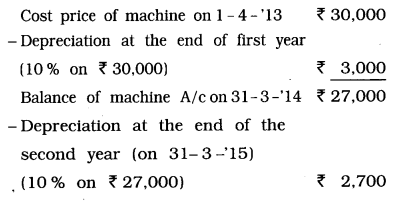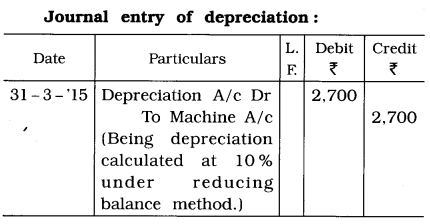3. On 1-4-’14 book value of machine was 34,000. Depreciation is calculated at 20 % under reducing balance method. This
machine was sold at 35 % profit on book value on 30-9- ‘14. From this information write journal entry for sale of machine.
Ascertain selling price of machine:
Book value of machine on 1 -4-’14 ₹ 34,000
– DepreciatIon at 20 % for 6 months
up to 30-9-’14= $$34,000 \times \frac{20}{100} \times \frac{6}{12}$$ = ₹ 34,000
∴ Book value of machine on 30-9-’14 ₹ 30,600
+ 35 % Profit on ₹ 30,600
= $$=30,600 \times \frac{35}{100}$$ = ₹ 10,710
∴ Selling price of machine ₹ 41,310

Journal entry for sale of machine:4. Hiral Limited charges depreciation on Its assets at 5 % under straight-line method. If annual depreciation amount is 6,000,
determine cost price of machines.
Cost price of machines:
Annual depreciation D = $$\frac{C \times R}{100}$$
∴ Cost price of machines can be obtained as follows:
Where, D = 6,000; R = 5 and C=?
Cost price of machines C = $$\frac{D \times 100}{R}$$
= $$\frac{6,000 \times 100}{5}$$
= 1,20.000
∴ Cost price of machines = ₹ 1,20.000

5. Sarvesh Limited has purchased a machine on 1 -4- ‘13. By charging depreciation at 10 % under reducing balance method, ₹ 3,240 is recorded as depreciation on 31 -3 -‘15, determine cost price of machine.
Cost price of machine: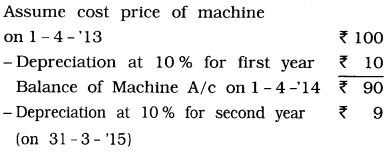Here, amount of depreciation on 31 -3- ‘15 is gIven ₹ 3,240.= $$\frac{3,240 \times 100}{9}$$ = ₹ 36,000
∴ Cost price of machine = ₹ 36,000Question 4.
Answer the following questions to the point:

1. ExplaIn the meaning of depreciation and describe u.s characteristics.
Depreciation: Generally, depreciation means reduction in the value of an asset.
Depreciation is the amount written oil from the value of an asset during it’s useful life.
Meaning/Definitions:
1. William Pickles: A gradual and permanent reduction in the quality, quantity and value of an asset is called depreciation.
2. Carter: Depreciation is the gradual and permanent decrease In the value of an asset from any cause.
Characteristics of depreciation: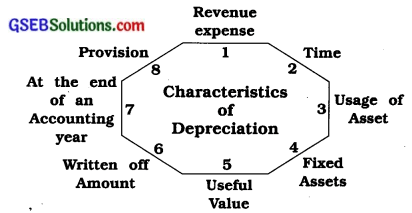1. Revenue expense: Depreciation is a revenue expenditure. So it is debited to Profit and Loss Account.
2. Time: Depreciation is an expense associated with time.
3. Usage of asset: Depreciation is based on consumption along with time.
4. Fixed assets: Depreciation is to be calculated on fixed tangible assets of a business.
5. Useful value: Depreciation indicates continuous and permanent reduction in the useful value of assets.
6. Written off amount: Depreciation is not payable in cash, hence, the amount written off as depreciation during a year remains in the business.
7. At the end of an accounting year: Generally depreciation is calculated at the end of an accounting year.
8. Provision: Depreciation is one kind of provision.

2. What are the objectives for charging depreciation?
Depreciation is an expense like any other revenue expense. But it is not paid in cash. In spite of this, it is taken into consideration while preparing accounts due to the following reasons :1. To find out true and fair profit: In order to know the true and fair profit or loss of the business, all expenses and incomes should be considered. Depreciation is the reduction in the value of the asset and thus shows a part of the consumed asset. So, it is a revenue expenditure and without considering this, a true picture of profit or loss will not be available. In short, it is essential to consider depreciation to know the true and fair profit-loss of the business. Thus, it is debited to Profit and Loss Account.

2. To know the true and fair financial position: If the assets of the business are shown at a price less than their depreciation in the Balance Sheet, then a true and fair financial position will be indicated. If depreciation is not deducted from the concerned asset, the Balance Sheet will not reflect a true position of financial affairs.

3. Replacement of the asset: In any business, if the value of the asset decreases after a specified period, it becomes necessary to acquire or purchase a new asset to replace the old one. For this, a provision for depreciation is made to keep the capital intact. Normally, the amount transferred in this, maybe invested in some government or gilt-edged securities. At the time of replacement of an asset, the amount will be realised by selling such securities. Thus, it is not required to raise new capital as new asset can be purchased from such provision.

4. To determine the true cost of production: In order to determine the cost of goods produced in factory or services, expense affecting production or production-related expenses should be considered. Thus, like other expenses depreciation on depreciable assets used in business is essential to be considered to determine the cost of goods. If depreciation, being an expense is not taken into account, then the true cost of the goods produced cannot be determined.

5. To comply with legal provision: According to section 205 of Companies Act, no company can distribute dividends without making a provision for depreciation from its profits. It is prudent to provide for depreciation from the viewpoint of sound accounting principles.

6. To consider as a paid business expense:
The revenue expenses of a business are based on time. Depreciation too is a revenue expense based on time. Depreciation is also pre-paid expense of business. Therefore, it is written off proportionately at the end of each accounting year as depreciation. Hence, depreciation should be considered as a paid business expense.

7. Determination of selling price: Profit of business is based on selling price of goods or services. And selling price of goods or services is based on cost of goods or service. Therefore, to arrive at a selling price of goods or services, total cost is determined, where depreciation is included.

3. Define depreciation and discuss factors of depreciation.
Definitions:
1. R. G. Williams: The gradual diminution in the value of an asset due to wear and tear, effluxion of time or new invention is termed as depreciation. It may be defined as a gradual depreciation in the value of asset due to use.

2. Matchman and Slawine: Depreciation is a process of estimating and recording a periodical charge arised because of the exhausted useful life of capital assets.

Factors of depreciation: followings are the factors or causes of depreciation :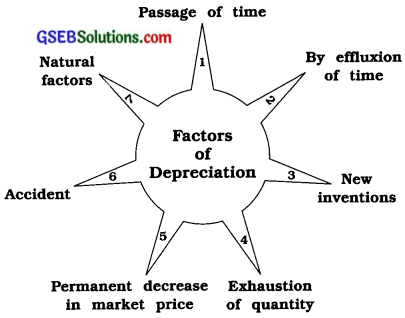1. Passage of time: With the usage and consumption, the asset depreciates and its useful life also decreases. With more usage, its useful life will decrease to a greater extent, e.g., Machinery will be more depreciated if it is used in three shifts.

2. By effluxion of time: Life of some of the assets ultimately becomes zero at the end of a specified period, e.g., For a land on lease, its value will be zero at the end of a specified period. Here, it is immaterial whether the asset is used or not.

3. New inventions: Sometimes, due to new technology and inventions, the assets in use become useless or less profitable. In such a case, a new asset will be purchased to replace the old one. Thus, a possibility of reduction in the useful life of the asset because of new inventions should be taken into consideration as a factor of depreciation.

4. Exhaustion of quantity: The value of some asset is due to the quantity contained in it. e.g., a mine or an oil well. In such cases, assets get slowly exhausted and a day comes when it gets completely exhausted and has to be abandoned. This reduction is known as depreciation.

5. Permanent decrease in market price: When the market price of any asset shows a permanent reduction in price as compared to its cost price, such reduction should be considered as depreciation of an asset.

6. Accident: Sometimes the assets meet with an accident. Such an accident causes damage to the asset which reduces the efficiency and utility of the asset.

7. Natural factors: The natural factors like flood, earthquake, rain, etc. reduce the useful life of the asset and thus decrease its efficiency as well as its cost. It is a type of depreciation. When quantity of petrol, spirit, etc. diminishes due to evaporation, it is considered as depreciation.

4. Explain the factors in detail to be kept in mind to determine annual amount of depreciation and rate of depreciation.
The following points are to be considered while ascertaining the amount of depreciation and the rate of depreciation :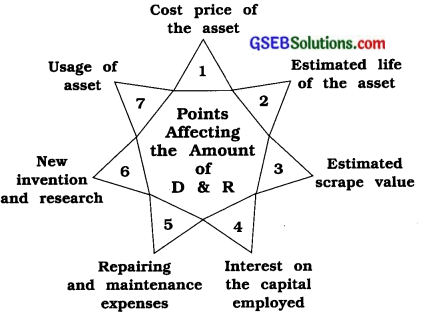1. Cost price of the asset: While determining the cost price and the purchase price of the asset, all expenses incurred to bring it up to the place of its use, its erection and installation charges are taken into consideration. In the case of old assets, if repairing is necessary, all expenses incurred on it, including the repairing expenses should be added to the purchase price.

2. Estimated life of the asset: The estimated life of the asset is the estimated period of time during which the purchased asset can be used effectively. While determining the estimated life, all the technical facts, capacity of the assets, possibilities of new inventions and business experience, etc. are to be considered.

3. Estimated scrap value: At the end of its useful life, the asset became useless. While calculating the rate of depreciation, the estimated scrap value is to be considered and deducted from the original cost of the asset.

4. Interest on the capital employed: When an asset is purchased, some capital is invested in it. If this capital was invested outside the business, it would have earned some interest. Thus, while calculating depreciation, such interest is to be taken into consideration.

5. Repairing and maintenance expenses: If money is spent on repairs and maintenance of the asset, its useful life can be increased. So, while calculating depreciation, such aspects should be considered.

6. New inventions and research: In order to face the business competition, it becomes necessary to acquire new modern machines. For this, it is necessary to know about the possible new inventions in the assets used in the business and their time interval. While determining the amount of depreciation, this time interval is considered.

7. Usage of asset: The usage of the asset is an important factor in determining the rate of depreciation. If the usage of asset is more, the amount of depreciation will be more. If a machine in the factory is used for more than one shift, the depreciation should be calculated more.5. What are depreciable assets? Give its explanation and list out depreciable assets.
Meaning: Depreciation is calculated on the fixed tangible asset of a business, so such assets are known as depreciable assets. There is reduction in value of some assets due to their limited life, they are also called as depreciable assets.

Explanation: In normal circumstances, the following explanation can be given for depreciable assets :
1. An asset which has limited life, such asset is called as depreciable asset, e.g., Furniture, Machine, Vehicles, etc. But asset which has unlimited life, this kind of asset is not considered as depreciable asset, e.g., Land.
2. An asset which is purchased for the purpose of business use, it means, which is not purchased for sale, such asset is called as depreciable asset, e.g., Furniture and fixtures, A.C. machine, Motor-car, etc.
3. An asset which is used for more than one accounting year, such asset is called as depreciable asset.

List of depreciable assets: The list of depreciable assets is as follows :

• Plant and Machines;
• Furniture and fixtures;
• Building;
• Leasehold properties;
• Vehicles;
• Mines, Oil wells and gas wells;
• Loose tools.

6. Write a short note :

1. Equal instalment method
Here, the scrap value of the asset is deducted from the cost price. Then, that amount is divided by the number of years of estimated useful life of the asset. The figure so arrived is the annual depreciation under equal instalment method. This method is also known as straight-line method or fixed instalment method. Under this method, the amount of annual depreciation remains identical.

Procedure to determine the amount of depreciation:
This is very simple and widely used (popular) method to determine the amount of depreciation. The annual amount of depreciation can be obtained with the help of the following formula :
(1) Annual Depreciation D = $$\frac{\mathrm{C}-\mathrm{S}}{\mathrm{N}}$$
(2) Annual Depreciation D = $$\frac{C \times R}{100}$$
Where, D = Depreciation
C = Cost price
S = Scrap value
N = Total number of years of useful life
R = Rate of depreciation

Features :

• Depreciation is calculated on the cost price of the asset.
• The amount of depreciation for each year remains equal.
• The graph of depreciation of each year remains in horizontal line.
• The depreciation amount is/fixed every year.

1. It is an easy method to understand.
2. The method of calculating depreciation is easy.
3. As the depreciation amount is the same every year, it is not required to be calculated repeatedly.
4. At the end of the useful life, the value of the asset becomes zero or equal to its scrap value.

• The amount of depreciation remains the same every year till the end of its useful life, whereas the repairs go on increasing as the asset becomes older.
• This method becomes complicated in case of assets which are frequently purchased and sold.
• This method does not consider the interest on capital blocked up in the asset.
• Here, no sufficient provision is made for the replacement of the asset.
• A proper balance cannot be maintained between the depreciation and the repairing expenses because in the initial years, the repairing expenses are less.
• While taking depreciation, only time factor is taken into consideration, e.g., the depreciation on the machines used in one shift is the same as the machines used in two shifts.

2. Reducing balance method
In this method, the amount of depreciation will be calculated at fixed rate on the opening balance of the asset every year. It is not calculated on the cost price of the asset. The amount of depreciation goes on decreasing every year. Thus, this method is known as reducing balance method.

Formula for calculating the amount of annual depreciation:
Annual depreciation D = $$\frac{\mathrm{C} \times \mathrm{R}}{100}$$
Where, C = Cost price (or the opening balance for that year, from the second year)
R = Rate of depreciation
D = Amount of annual depreciation
Under this method, the amount of depreciation is determined at the stipulated rate of depreciation. The amount depreciation for the first year is calculated on the cost price of the asset and thereafter every year it is calculated on the opening balance of the asset of that year.

Features:

• Every year the amount of depreciation goes on decreasing.
• The total amount of the cost price of the asset is never written off to the full extent, and so the cost price of the asset will never be zero at any time.
• There is a balance between depreciation amount and repairing charges.

Suitable for which assets: This method is suitable for assets which have a long or indefinite useful life. This method is suitable to the assets like: Plant and Machinery, furniture, etc. For e.g., The depreciation of 10% on an asset of ₹ 2,000 will be ₹ 200 in the first year. The opening balance of the second year will be ₹ 1,800 and again 10% on this will be ₹ 180 which is the depreciation for the second year. In the third year, depreciation will be calculated on ₹ 1,620.

• Here, the amount of depreciation is higher in the starting years and decreases in the later years. The repairing charges are nil in the starting years and higher in the later years. So, there is a balance between the two.
• This method is more logical, scientific and reasonable.
• This method is adopted for purpose of Income Tax in India.
• The total amount of the cost price of the asset is never written off to its full extent and so it never becomes zero.

• The interest on capital is not taken into consideration.
• Calcualtion of depreciation is not easy.
• There is no provision for the replacement of the asset.
• If there is a mistake in calculating the depreciation, then the true profit or loss and true financial position of business will not be revealed.7. Give difference: Equal Instalment Method and Reducing Balance Method

 Point Equal Instalment Method Reducing Balance Method 1. Calculation of the amount of depreciation The annual amount of depreciation is available by dividing the cost price (C) less scrap value (S) by number of years of useful life (N). Formula: D = $$\frac{C-S}{N}$$ At a fixed rate (R), depreciation is calculated on the opening balance of the asset every year (C). Formula: D = $$\frac{\mathrm{C} \times \mathrm{R}}{100}$$ 2. Amount of depreciation In this method, amount of depreciation remains equal in all years. In this method, the amount of depreciation goes on decreasing every year. 3. Repeated calculation As the depreciation remains equal, there is no need to calculate depreciation again and again. Calculation of depreciation is required every year as it changes every year. 4. Value of asset at the end of estimated life While charging depreciation, the whole cost price of the asset can be written off and the asset value becomes zero at the end of its estimated life. While charging depreciation, the whole cost price of the asset is not written off and the asset value never becomes zero at the end of its estimated life. 5. On which price depreciation is calculated? Depreciation is calculated on the cost price of the asset every year. Depreciation is calculated on the book value of the asset every year. 6. Repairing expenses No proper balance between the amount of depreciation and repairing expenses is maintained. Proper balance can be maintained between the depreciation amount and repairing expenses. 7. Suitable for which assets. If the life of the asset is certain and short, this method is suitable. If the life of the asset is long, this method is suitable. 8. Ease of calculation Calculation of depreciation is easy in this method. Calculation of depreciation is not easy in this method.

8. Explain, different methods for recording of depreciation in books of accounts.
From the viewpoint of accounting entries, there are two main methods of recording depreciation in books of accounts :
1. Method to write-off the depreciation directiy to the concerned asset account.
2. Method to create the provision for depreciation account.

Explanation of both the methods with illustration are as under:
1. Method to write-off the depreciation directly to the concerned asset account: Under this method, depreciation is calculated on an asset by the stipulated method and is debited to depreciation account and credited to the asset account. As a result, the book value of the asset goes on reducing by the amount of depreciation.

Illustration: Ashok Limited has purchased furniture for ₹ 50,000 on 1-4-’15. 10% depreciation is to be charged as per straight-line method on this furniture. Pass journal entries for first two years and disclose effect in annual accounts in the books of company.
Journal Entries of Ashok Limited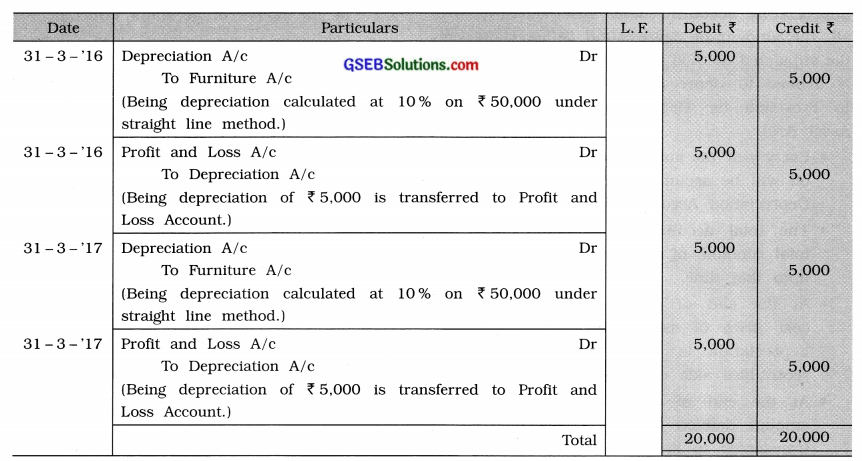Note: As shown above, at the end of every accounting year (i.e., on 31-3-’16 and on 31-3-’17) the amount of depreciation will be transferred to Profit and Loss Account.Note: As shown above, the asset (Furniture) is shown in Balance Sheet at the value derived after deducting the depreciation of that year from Its book value.2. Method to create the provision for depreciation account: Under this method, first of all, the amount of depreciation is ascertained by the stipulated method. The amount of depreciation is debited to Depreciation Account and credited to Provision for Depreciation Account (not to Asset A/c).

• Every year the amount of depreciation written off will be accumulated in the Provision for Depreciation Account.
The total accumulated amount shows the total amount of depreciation on that asset up to that time.
• At the end of each accounting year, the cost price of assets less total accumulated depreciation is shown on the asset and receivables side of the Balance Sheet.
• At the end of each accounting year, the amount debited to Depreciation Account is transferred to Profit and Loss Account.
• Provision for Depreciation Account is reflected in the books of accounts until the asset is sold off.
• As the annual depreciation is accumulated in the Provision for Depreciation Account, this method is also known as the method of Accumulated Depreciation.

Illustration: Chintan Limited has purchased a machine on 1-4-’15 for ₹ 50,000 by cheque. Provide depreciation at 10 % by fixed instalment method by creating provision for Depreciation Account. Prepare the journal entries of the company for the first two years and show the effects in the final account and prepare Provision for Depreciation Account and Machine Account.
Calculation of annual depreciation:
D = $$\frac{\mathrm{C} \times \mathrm{R}}{100}$$ = $$\frac{50,000 \times 10}{100}$$ = 5,000
∴ Annual depreciation is ₹ 5,000.
Journal Entries of Chintan Limited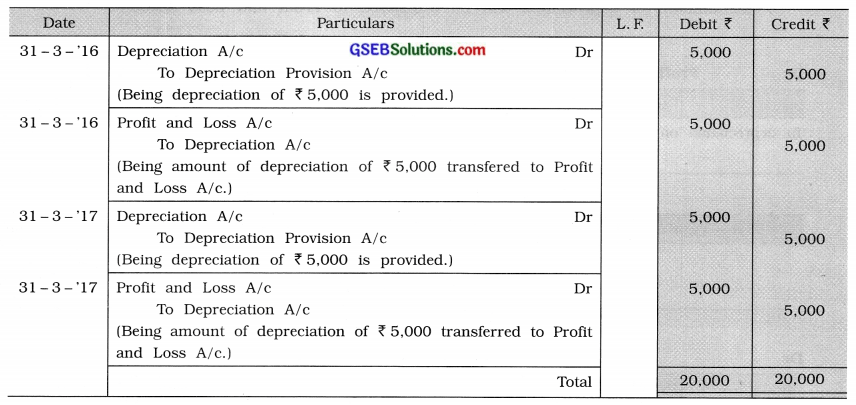Ledger of Chintan Limited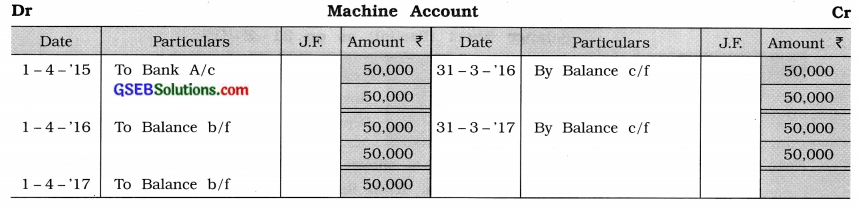Depreciation Provision Account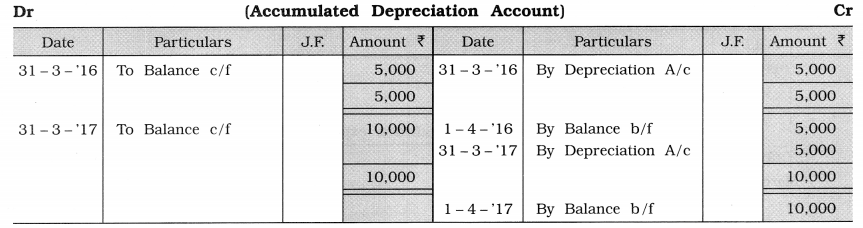Accounting effect in Annual AccountsQuestion 5.
Answer the following questions as directed :

1. Suresh Limited has purchased machine for ₹ 63,600 on 1-4-’12. Installation expense of machine was incurred ₹ 1,000. If scrap value is estimated to be ₹ 14,600 after its estimated life of 8 years, determine annual amount of depreciation and annual rate of depreciation. Accounting year of company ends on 31st March. Write journal entries for first three years and also prepare machine account and depreciation account. Show accounting effect in annual accounts of first year. Company charges depreciation under straight line method of depreciation.
Annual depreciation D = $$\frac{\mathrm{C}-\mathrm{S}}{\mathrm{N}}$$
Where, D = Amount of annual depreciation = ?
C = Cost price
₹ 63,600 + ₹ 1,000 = ₹ 64,600
S = Scrap value = ₹ 14,600
N = Total useful life in years = 8 years
∴ D = $$\frac{64,600-14,600}{8}=\frac{50,000}{8}$$ = 6,250
∴ Amount of annual depreciation = ₹ 6,250 …………………….. (1)
∴ Annual rate of depreciation (R) = $$\frac{\mathrm{D} \times 100}{\mathrm{C}}$$
= $$\frac{6,250 \times 100}{64,600}$$
= 9.674 %
∴ Annual rate of depreciation (R) = 9.674 % ………………….. (2)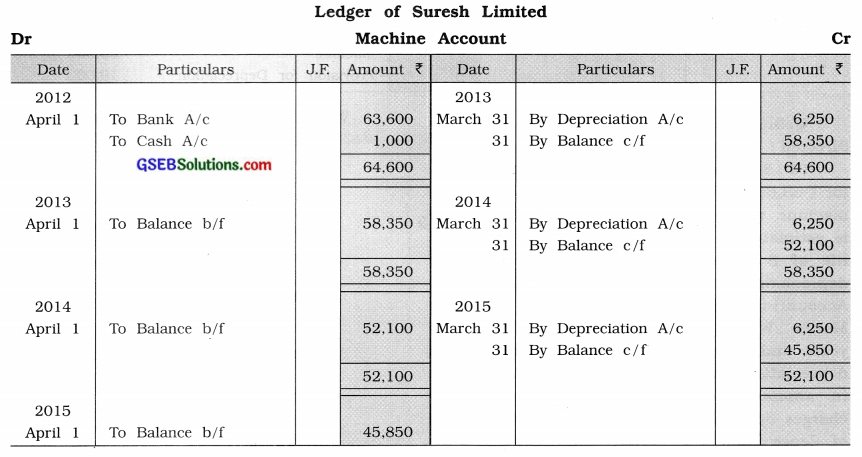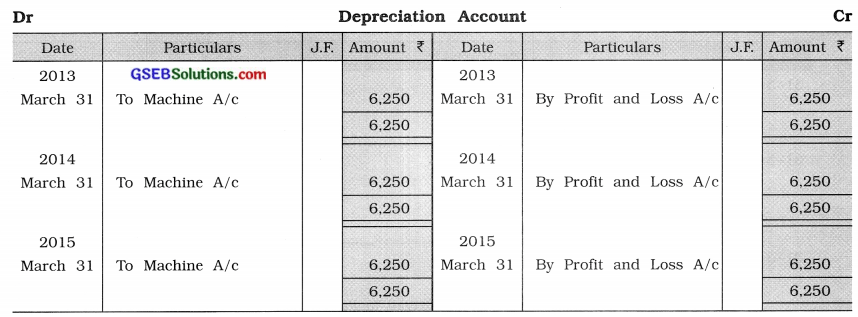2. On 1-4-’13 Hansa Limited has balance in machine account in its books of ₹ 36,000. This machine was purchased seven years back. This machine was sold at 30 % profit of its book value on 31-3-’14. Every year company charges depreciation at 10% under straight line method.
From the above information pass necessary journal entries and prepare machine account.
Under straight line method, the amount of depreciation is determined on the basis of cost price of asset. Therefore, first of all, cost price of machine (asset) will be ascertained.As given, book value of machine at the end of seventh year is ₹ 36,000∴ Cost price of machine = ₹ 1,20,000
∴ Annual depreciation at 10% of ₹ 1,20,000 = ₹ 12,000

Selling price of machine:Journal Entries of Hansa Limited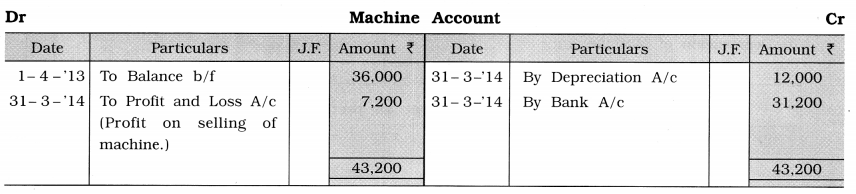3. On 1-4-12 Harun Dodawala Industry has purchased machine for ₹ 2,75,000. The installation expense of this machine was ₹ 15,000 and carriage was ₹ 10,000. Company provides depreciation at 8 % every year under straight-line method. This machine was sold at 20 % loss of book value on 31-3-’15. Pass journal entries for three years and machine account for three years.
Cost price of machine C = Purchase price + Installation expense + Carriage expense
= ₹ 2,75,000 + ₹ 15,000 + ₹ 10,000 = ₹ 3,00,000
Company provides depreciation at 8 % every year under straight line method.
Annual depreciation D = $$\frac{\mathrm{C} \times \mathrm{R}}{100}=\frac{3,00,000 \times 8}{100}$$ = ₹ 24,000

Selling price of machine:Journal Entries of Harun Dodawala Industry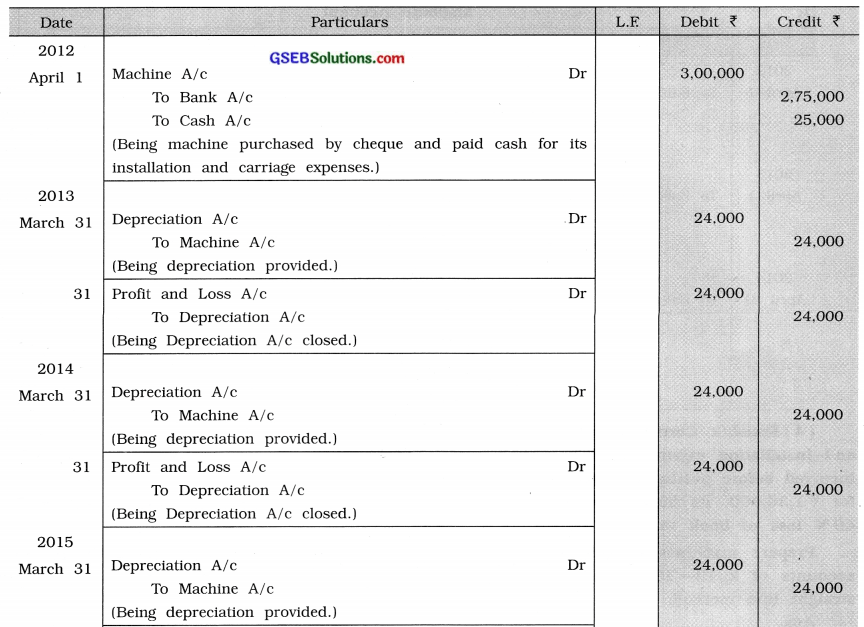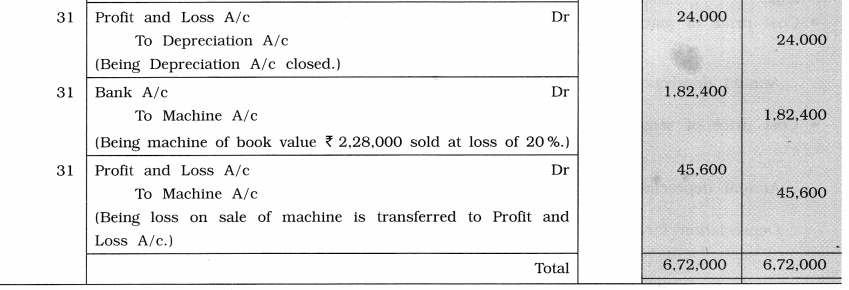Ledger of Harun Dodawala Industry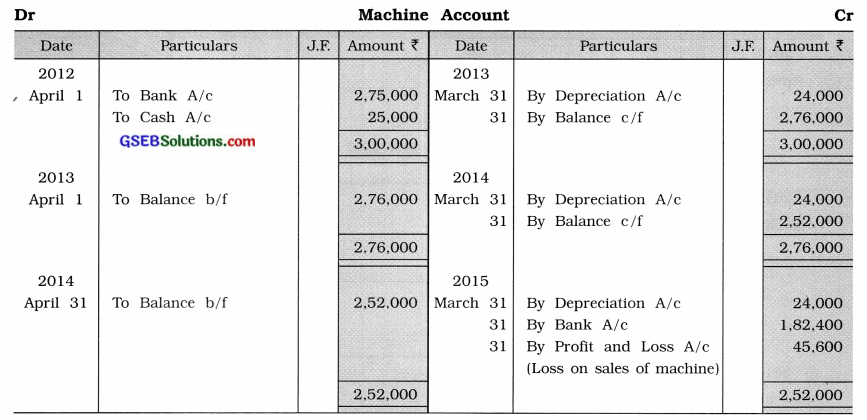4. Kaushik Corporation has purchased machine for ₹ 1,24,000 on 1-4-’12. The carriage and installation expense of this machine was ₹ 16,000. The repairing expense of ₹ 20,000 was incurred before putting this machine for production. On 1-10-’13 second machine was purchased for ₹ 1,36,000, its installation expense was of ₹ 8000. First machine was sold on 30-9-’14 at 40 % loss of book value.
Prepare machines account up to 31-3-’15 and show its accounting effect in the annual accounts of 2012-’13. Company provides depreciation on machines at 10% every year under straight-line method.
Cost price of machine on 1 -4-’12 = ₹ 1,24,000 + ₹ 16,000 + ₹ 20,000 = ₹ 1,60,000
Annual depreciation on first machine = $$₹ 1,60,000 \times \frac{10}{100}$$ = ₹ 16,000

Cost price of second machine purchased on 1 – 10 -13 = ₹ 1,36,000 + ₹ 8,000 = ₹ 1,44,000
∴Annual depreciation on second machine = $$\text { ₹ } 1,44,000 \times \frac{10}{100}$$ = ₹ 14,400
∴ Depreciation for six months from 1- 10-’13 to 31-3-14 = $$\text { ₹ } 14,400 \times \frac{6}{12}$$ = ₹ 7,200

Selling price of first machine: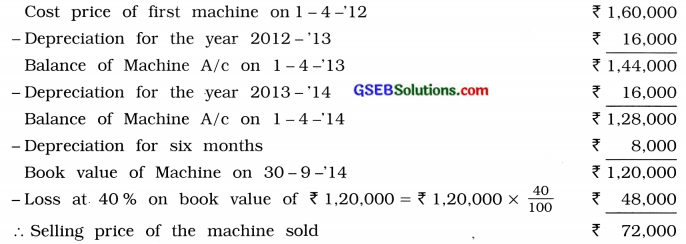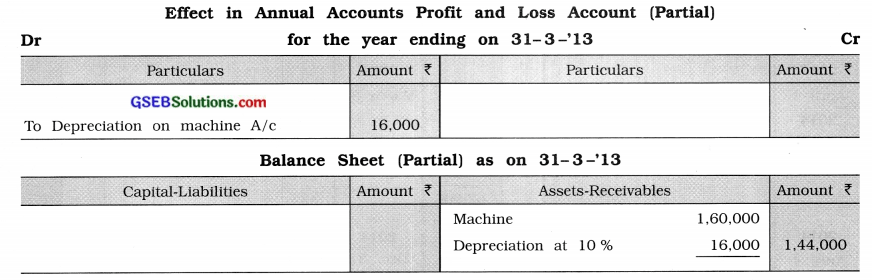5. Machine account shows balance of ₹ 60,000 in the books of Mehta Limited on 1-4-’12. This machine was purchased five years back. Till today, total depreciation written off on this machine was of ₹ 20,000. On 1-1-’14 second machine was purchased for ₹ 12,000.

Second machine was not found suitable and sold at 10% loss of book value on 31-12-’14. Every year company provides depreciation at 5 % under straight-line method.’
From the above information prepare machine account and depreciation account in the books of company up to 31-3-’15.
Here total depreciation of 5 years is given ₹ 20,000. Divide this depreciation amount with 5 years, annual depreciation will be received.
Annual depreciation under straight line method == $$\frac{20,000}{5}$$ = 4,000
∴ Annual depreciation for first machine ₹ 4,000 ……………………….. (1)

Cost price of llrst machine before 5 years
= Book value of machine on 1 -4 -‘12 + Total depreciation charged for 5 years = ₹ 60,000 + ₹ 20.000 = ₹ 80,000
Cost price of second machine on 1 – 1 -‘14 ₹ 12.000
Annual depreciation for second machine at 5 % =$$₹ 12,000 \times \frac{5}{100}$$ = ₹ 600

Selling price of second machine: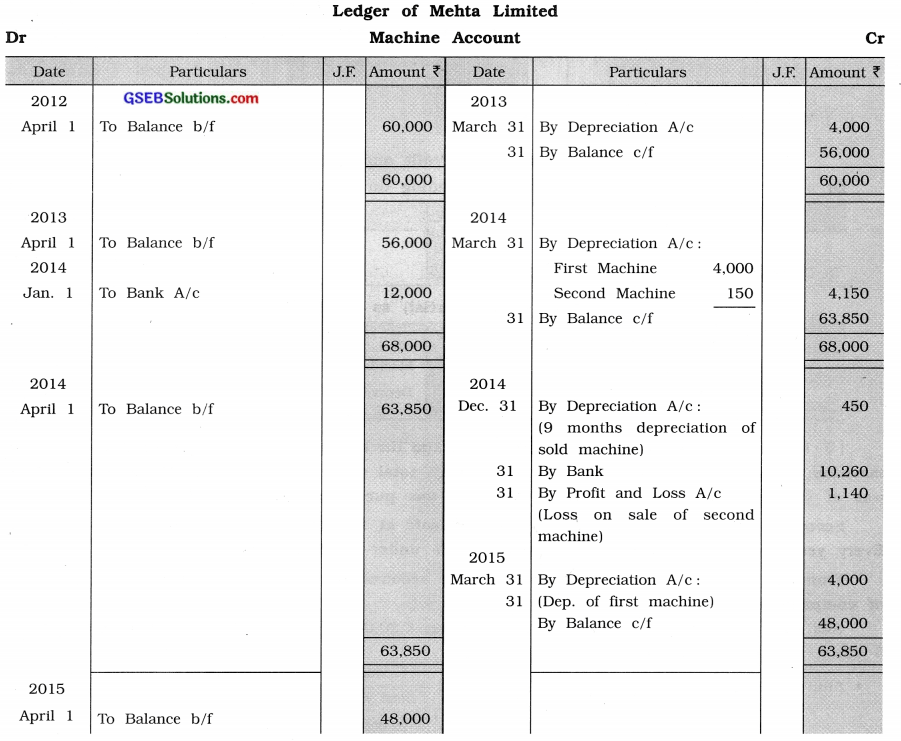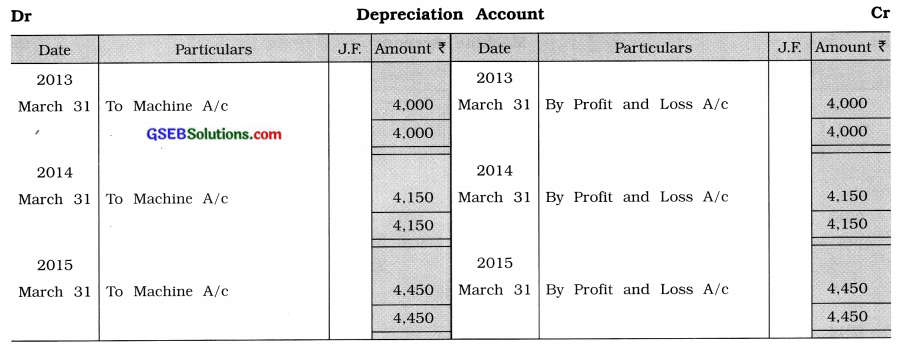6. Raj Kumar Limited had purchased some machines for 82,000 on 1-4- ‘12. The installation expense of this machine was of 8,000. On 1 – 10- ‘14 company purchased another machine for 70,000 and their installation expense was of 2,000. Company provides depreciation at 10 % every year under straight-line method. 30 % machines from first purchase were sold at 20 % profit of book value on 31-3-’15.
From the above information prepeare machines account up to 31 -3- ‘15 as well as show accounting effect in annual accounts of each year.
Cost price of first machinc on 1 – 4- ‘12 = ₹ 82,000 + ₹ 8,000 = ₹ 90,000
Annual depreciation = $$\text { ₹ } 90,000 \times \frac{10}{100}$$ = ₹ 90,000

Cost price of second machine on 1 – 10- ‘14 = ₹ 70,000 + ₹ 2,000 = ₹ 72,000
Annual depreciation = $$₹ 72,000 \times \frac{10}{100}$$ = ₹ 7,200
Six months depreciation from 1 – 10- ‘14 to 31 – 3- ‘15 = $$₹ 7,200 \times \frac{6}{12}$$ =₹ 3,600

Selling price of 30 % machine from first purchased machine:
Cost price of 30 % machine from first purchased machine of ₹ 90,000 = $$₹ 90,000 \times \frac{30}{100}$$
= ₹ 27,000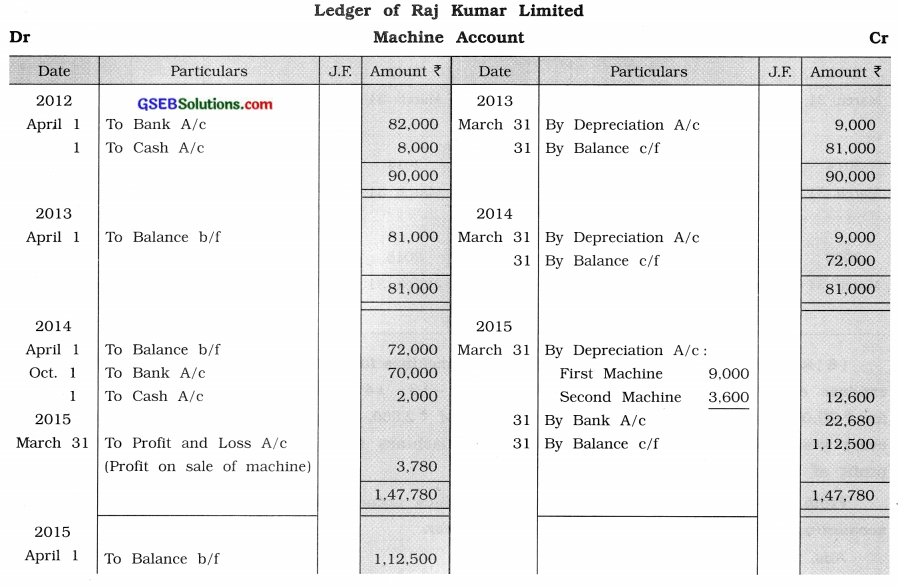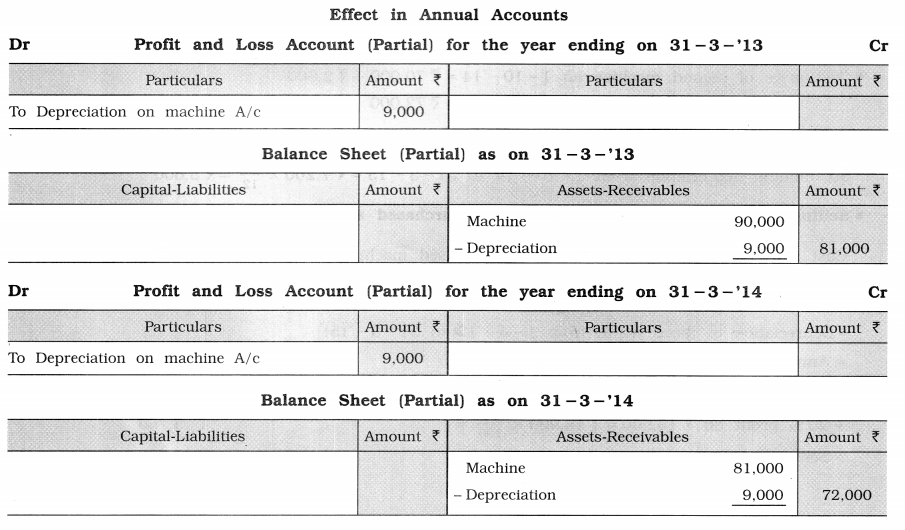7. Sharma Limited has purchased a machine for 67,000 on 1 -4 -‘12. Installation expense of this machine was ₹ 3,000. Depreciation is to be provided at 10 % every year under reducing balance method. Ascertain amount of depreciation, pass journal entries and prepare machine account and depreciation account for first three years. Show accounting effect in annual accounts of first two years.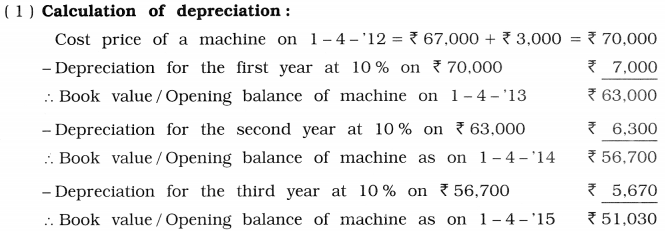(2) Annual depreciation is ₹ 7,000: ₹ 6,300 and ₹ 5,670 respectively.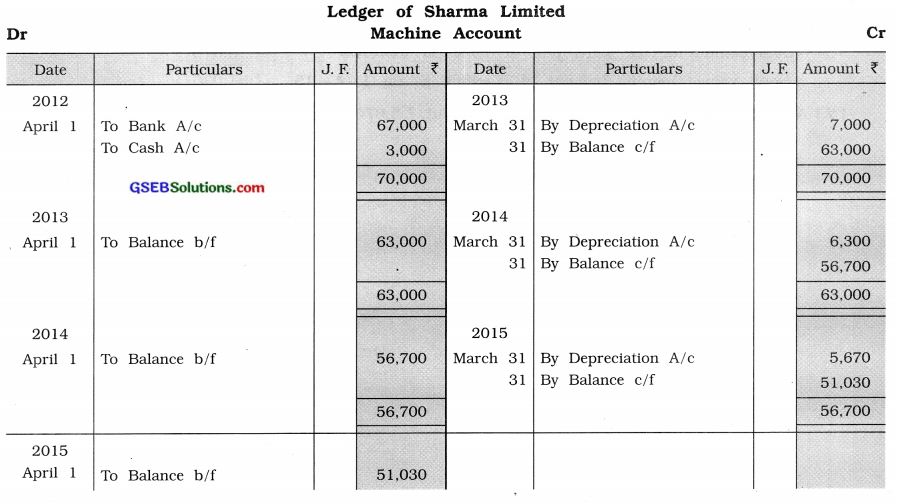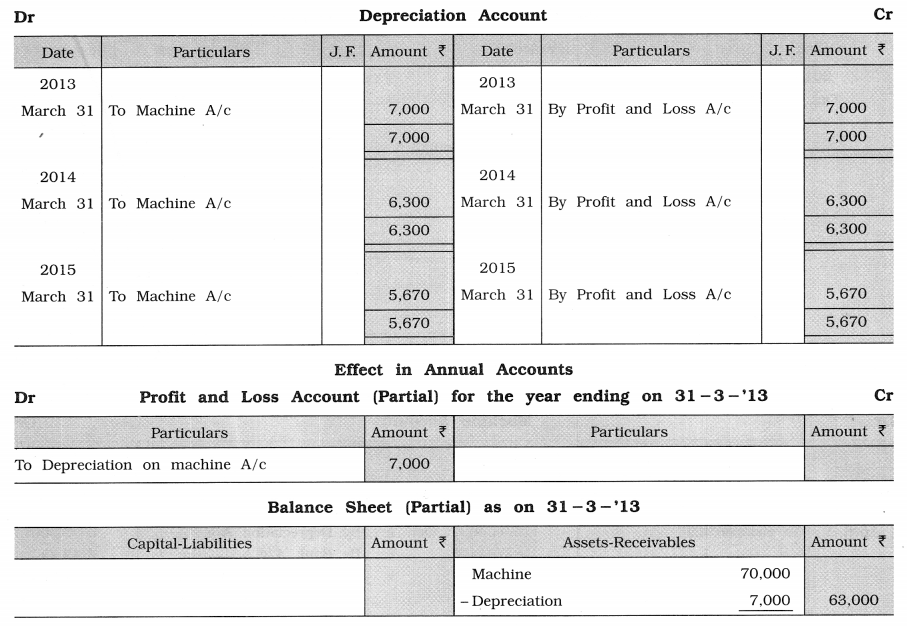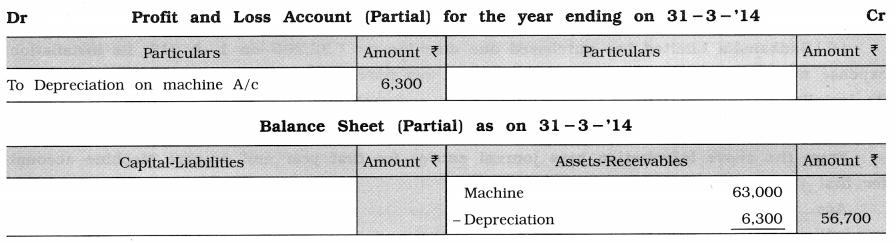8. Machine account shows balance of 60,000 in the books of Betul & Company on 1 -4 -‘14. Company provides depreciation at 10 % every year under reducing balance method. This machine was sold at 25 % loss on book value on 1 – 10- ‘14. From the above information pass necessary journal entries and prepare machine account in the books of company.
Selling price of the machine: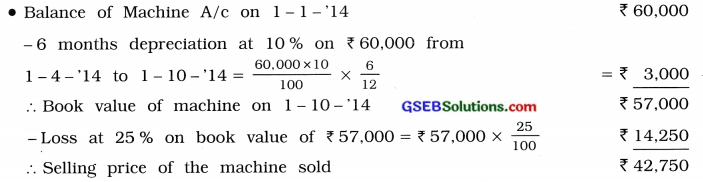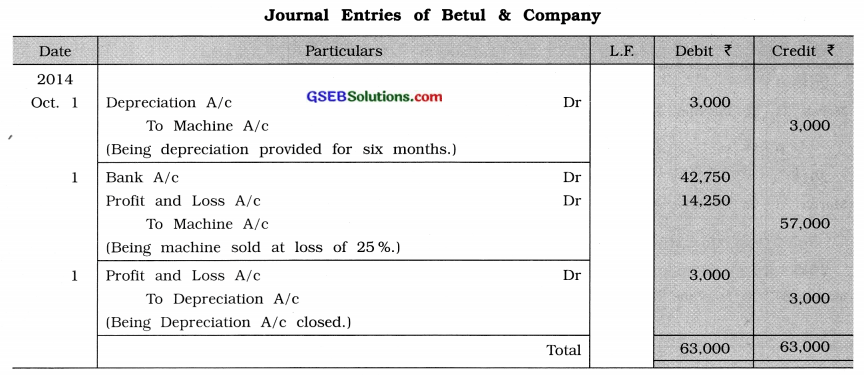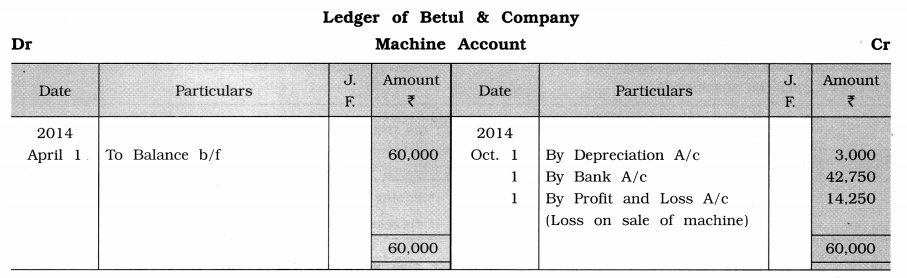9. Sudhanshu Limited has purchased one machine for 22,200 on 1 -4- ‘12, its installation expense was of 1800. Another second machine was purchased on 1 – 10- ‘13 for 20,400 and Its installation expense paid 1,200. Every year, company provides depreciation at 10 % under reducing balance method. On 31 -3- ‘15 first machine was sold at 20 % loss of its book value. From the above information pass journal entries for first year and prepare Machine account for first three years in the books of company.
Selling price of first machine: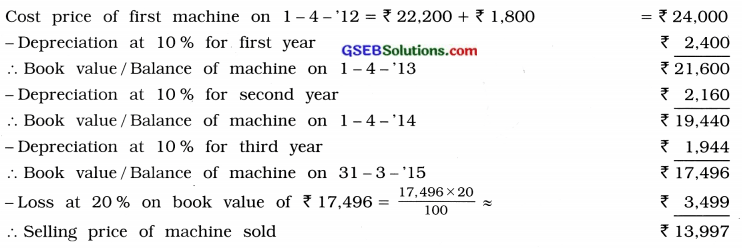Calculation of depreciation for second machine: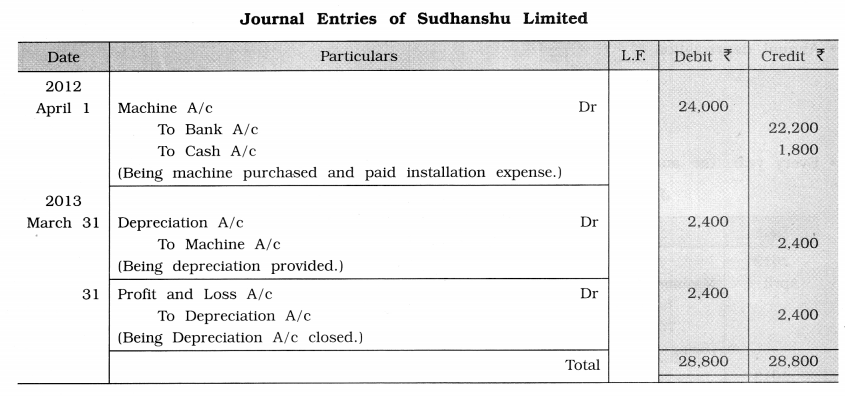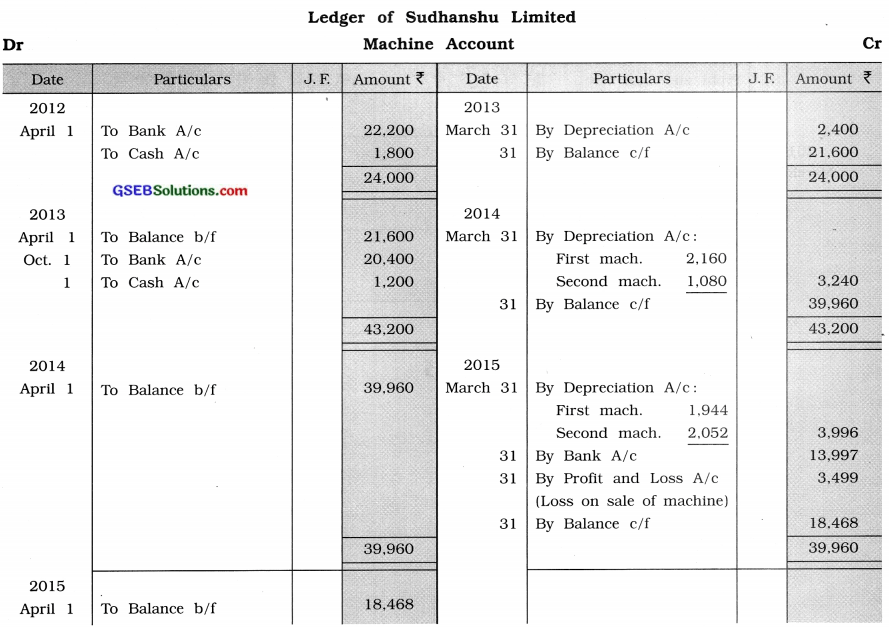10. Bhalchandra Manufacturing Limited has purchased one machine for ₹ 73,000 on 1-4-12. Its installation expense was of ₹ 2,000. It was decided to provide depreciation at 8 % under straight-line method. Company records depreciation by creating depreciation provisions.
From the above information pass journal entries, prepare depreciation provision account, depreciation account for first two years in the books of company.
Cost price of purchased machine on 1 – 4 – ’12 = ₹ 73,000 + ₹ 2,000 = ₹ 75,000
Annual depreciation D = $$\mathrm{C} \times \frac{\mathrm{R}}{100}$$
= $$₹ 75,000 \times \frac{8}{100}$$
= ₹ 6,000
Every year, the amount of depreciation under straight-line method remains the same ₹ 6,000
Journal Entries of Bhalchandra Manufacturing Limited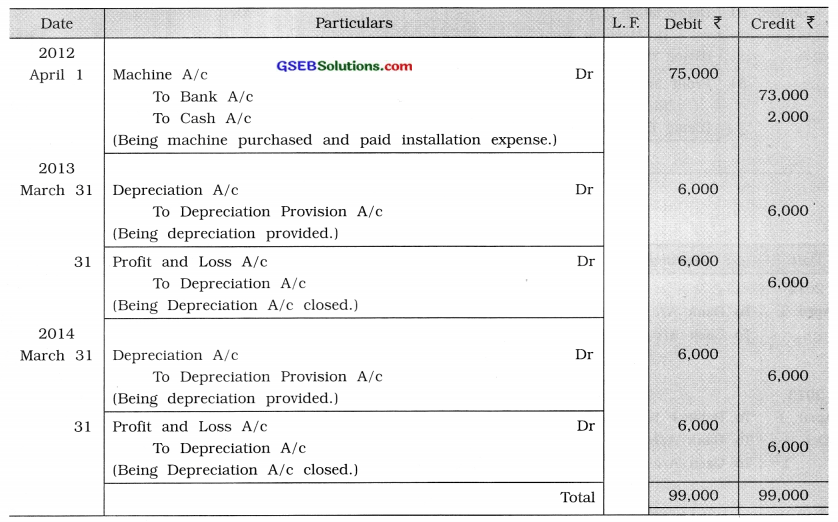Ledger of Bhalchandra Manufacturing Limited
Depreciation Provision Account11. Jenet Limited has purchased one machine for ₹ 78,000 on 1-4-’13. Its installation expense was of ₹ 2,000. On this machine depreciation is to be provided at 10% under reducing balance method. This depreciation is to be recorded by creating depreciation provision account (Accumulated depreciation account).
From the given information, pass journal entries of first two years and show its accounting effect in annual accounts of first two years in the books of company.